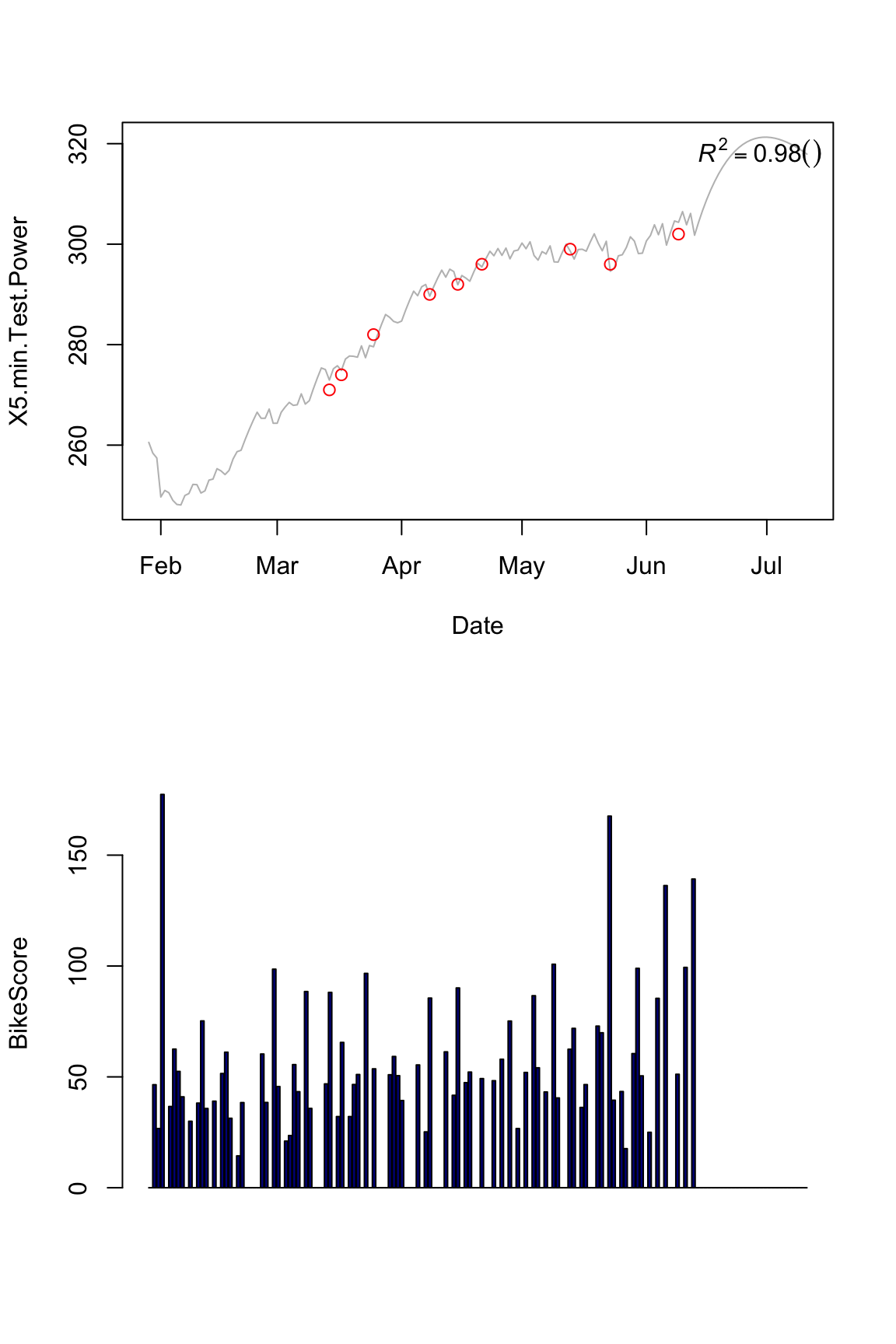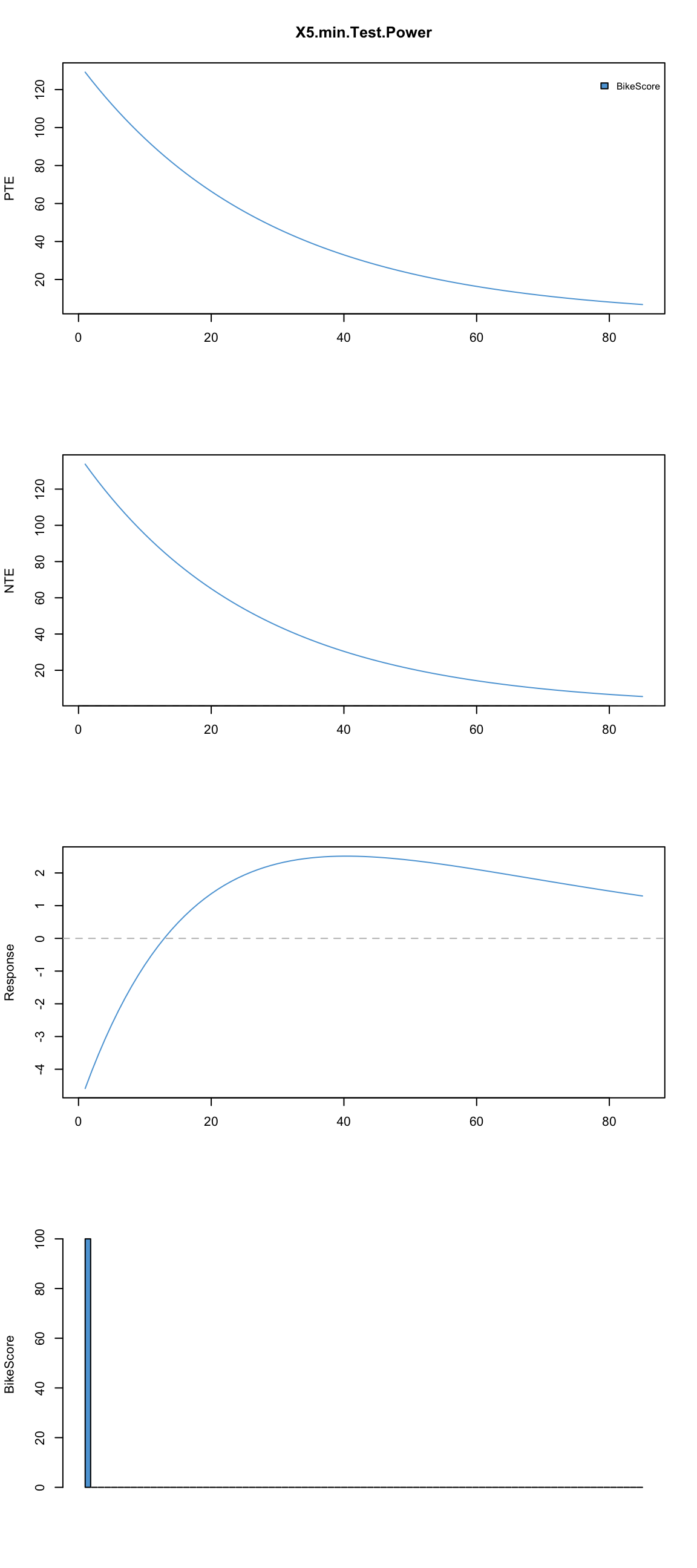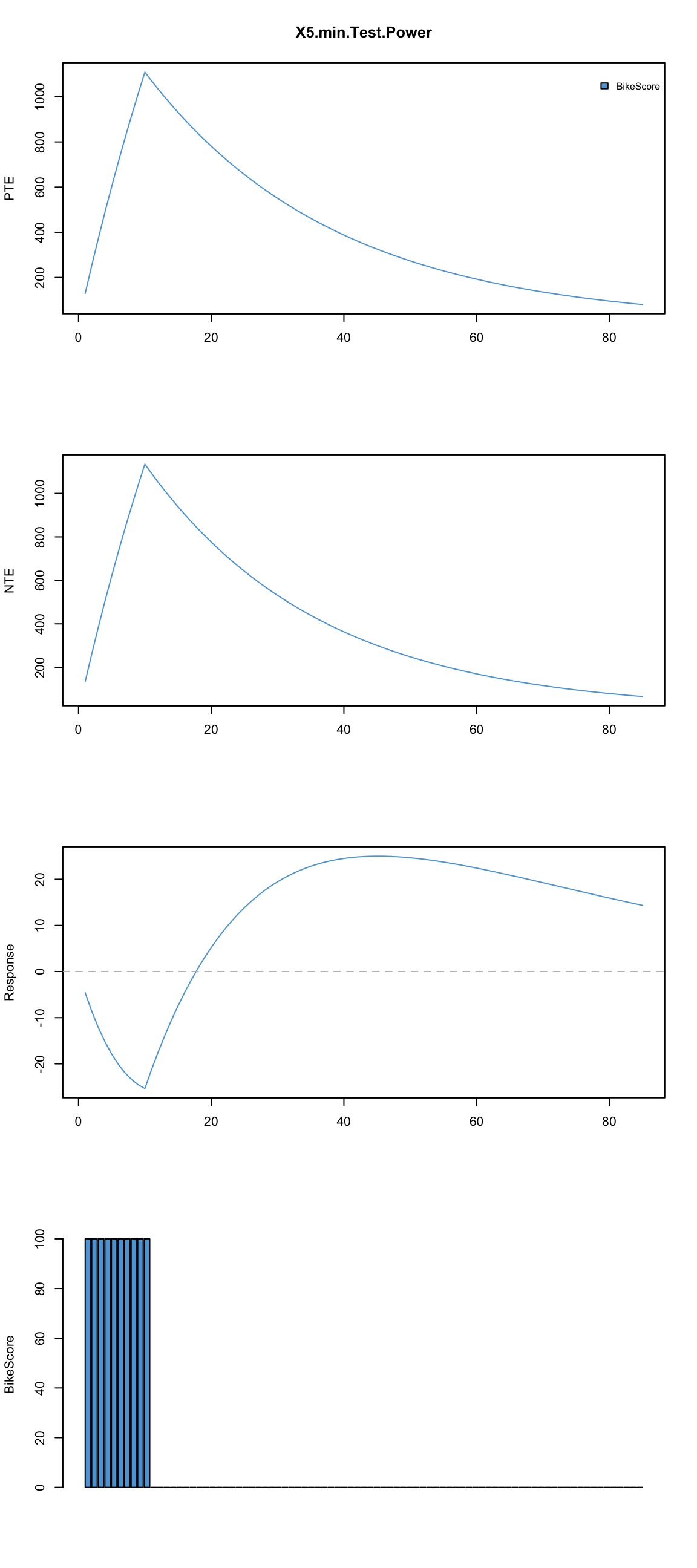In the previous part I’ve introuced multivariate modeling of impulse and response using Banister model. In this part I will continue with exploration of this model, mainly visualizing reaction predicted by the model on standardize impulse (load) and compare prediction using multiple impulses. I will use same data sets: one by Skiba and one randomly generated as in first installment.

Let’s load the Skiba data and train the model and plot it’s performance on training data set.

``````library(lubridate)

source("Banister Impulse Response Multivariate.R")

Skiba\$Date <- dmy(Skiba\$Date)
Skiba <- Skiba[1:3]
Skiba\$BikeScore[is.na(Skiba\$BikeScore)] <- 0

skiba.model <- train.Banister(data=Skiba,
impulse="BikeScore",
response = "X5.min.Test.Power",
dates = Skiba\$Date)

plot.banister(skiba.model)``````I have coded additional function to allow some some simple diagnostics of the model. For example, I am interested in how this athlete will react if I impose certain load on his, let’s say 100 units of Bike Score

``plot.banister.diag(skiba.model, standard.impulse = function(x) {100})``First plot is Positive Training Effect and second is Negative Training Effect. This plot is Response which is basicaly TPE - NTE assuming that p0 (starting performance) is equal to 0.

Bottom plot depicts impulse (or training load) in the bike score

As can be seen from the picture, single session of 100 units cause more fatigue (k2 > k1), but it lasts shorter (tau2 < taui1) hence we get that dip and supercompensation in the Response.

What if we repeat this 100s BikeScore points back to back for 10 days?

``plot.banister.diag(skiba.model, standard.impulse = function(x) {100}, impulse.loc = 1:10)``What if we evenly spread those 100s BikeScore points every 5th day?

``plot.banister.diag(skiba.model, standard.impulse = function(x) {100}, impulse.loc = c(1,6,11,16,21,26,31,36,41,46))``